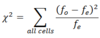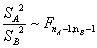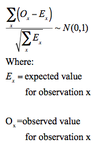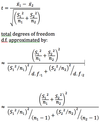# Tests Flashcards Preview

## Actuarial Science - General Maths & Stats > Tests > Flashcards

Flashcards in Tests Deck (13):
1

2

3

4

5

## How do you Do a One Way Analysis of Variance (ANOVA)?

### In order to compare the means of different groups of observations of random variables with a common variance, create ANOVA table and then: Calculate F ratio score and compare with  null hypothesis F score for significance level α given by: F0 = Fk-1, n-k, α  F is calculated as follows:6

7

## What is a chi-squared goodness-of-fit test, when should it be used?

### The goodness of fit chi-square tests are used to test whether a set of data (a sample) fits a stated probability distribution. The null hypothesis H0 is that the data fits the distribution, H1 that it does not. H0 is rejected if the chi-square score exceeds the critical value from the table for degrees of freedom ν, given by: ν = c - 1 - k where c is the number of categories for data, k is the number of population parameters estimated to calculate frequency. If graduating the data using standardised tables, drop a couple of degrees of freedom overall and then state this has been done. The chi-square statistic is the sum of squares of difference between observed and expected values, divided by the expected value:8

9

## How do You Test Whether the Variance of two Samples is Equal?

### If two samples, A and B, have nA and nB observations, with Sample Variances SA2 and SB2, then it is possible to test the null hypothesis H0 (variances equal) with a two-sided F-Test:10

## What is the Cumulative Deviations Test

### The Cumulative deviations test is a test for bias in data graduations12

## What is the t-test for comparing the means of two samples of unequal size from distributions with equal variance?

### t-test for samples of unequal size from distributions with equal variance13

## What is the t-test for comparing the means of two samples from distributions whose variances cannot be assumed to be equal?

### AKA Welch's t-test14